If you have a related question, please click the "Ask a related question" button in the top right corner. The newly created question will be automatically linked to this question.

# TPS38: TPS37/38 Design Calculator

Part Number: TPS38
Other Parts Discussed in Thread: TPS37

Hello team,

I have been using TPS37/38 Design Calculator for TPS38A.

https://dev.ti.com/gallery/view/LPVRS/TPS37_38_3760_Design_Calculator/ver/1.0.0/

1. What does it mean by Actual Reset Trigger? Is it TYP value of VIT+?
2. Why is the calculated result NaN? Do you see anything wrong in the setting?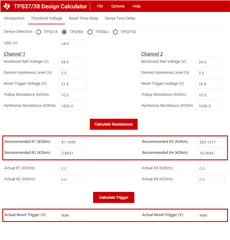Best Regards,

Kei Kuwahara

• Hi Kei,

The values for actual reset trigger in the calculator is determined by the values you put for the actual resistances on your board (R1, R2, R3, R4). The calculated resistances are likely not attainable with common resistor values, so the actual reset trigger calculation is provided to show the reset trigger thresholds with the resistor values that you actually use.

The image below shows the actual reset trigger values with R1, R2, R3, and R4 actually matching with the recommended values: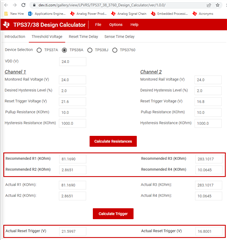And this image below shows the actual reset trigger values that you might get from using resistors that are close but not exact to the recommended R1, R2, R3, and R4 values: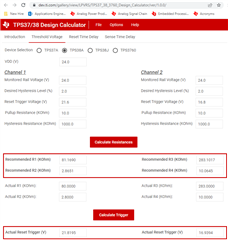TI Recommends keeping these values in mind for your design as the values you put in the calculator for reset trigger voltage are subjected to differences between your real resistor values and the recommended ones given by the calculator.

Please let us know if you have any questions,

Joshua Austria

• Hi Joshua-san,

Is the Actual Reset Trigger, TYP value or min/max?

Becuase the diagram shows VIT-(UV）MIN, VIT-(UV) TYP, VIT-(UV) MAX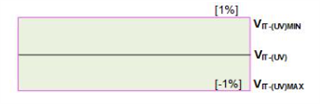And I have another question.

I have calculated Reset trigger voltage using this tool, but the result from the tool, and calculated value from the datasheet equation doesn't match.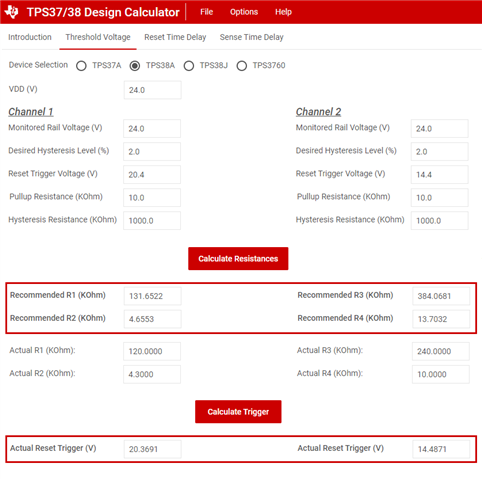The acutal reset trigger for R1=120kohm, R2= 4.3kohm is 20.3691V from the calculator tool.

However, from the datasheet equation 7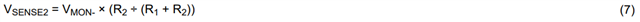Where VSENSE2 = 0.8V, R1=120kohm, R2= 4.3kohm

So, VMON- = 0.8V * (120k + 4.3k) / 4.3k = 23.126V

• The voltage difference is pretty big. What is the reason for this difference?
• Would it be possible to see the equation for the Actual Reset Trigger on the tool?

Best Regards,

Kei Kuwahara

• Hi Kei-san,

For the diagram you showed, the min and max values are subject to temperature, as temperature will modify VIT. The "TYP" value shown in the diagram is with ambient temperature (25 C), but the actual reset trigger can vary by around +/- 1% based on your temperature.

The tool will give a different result due to the hysteresis resistor. If the user is not using the hysteresis programming, then the hand calculation from equation 7 in the data sheet will provide the correct result. In the next revision of the calculator, an option will be available for this use case.

Hope this helps, and please let us know if you have any other questions,

Joshua Austria

• Hi Joshua-san,